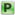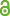Hauptmenü
• Autor
• Of, Günther
• Xuan, Thanh Phan
• Steinbach, Olaf
• TitelBoundary element methods for Dirichlet boundary control problems
• Datei
• DOI10.1002/mma.1356
• Erschienen inMathematical Methods in the Applied Sciences
• Band33
• Erscheinungsjahr2010
• Heft18
• Seiten2187-2205
• LicenceCC-BY
• Projekt Identifikator
• info:eu-repo/grantAgreement/FWF/SFB/F32/Mathematical Optimisation and applications in Biomedical Sciences/
• Zugriffsrechte• Download Statistik610
• Peer ReviewJa
• AbstractIn this paper we discuss the application of boundary element methods for the solution of Dirichlet boundary control problems subject to the Poisson equation with box constraints on the control. The primal and adjoint boundary value problems are rewritten as systems of boundary integral equations involving the standard boundary integral operators of the Laplace equation and of the Bi–Laplace equation. While the first approach is based on the use of the standard boundary integral equation based on the Bi–Laplace fundamental solution, the additional use of the normal derivative of the related representation formula results in a symmetric formulation, which is also symmetric in the discrete case. We prove the unique solvability of both boundary integral approaches and discuss related boundary element discretisations. In particular, we prove stability and related error estimates which are confirmed by a numerical example.